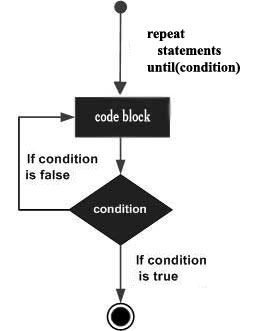# Lua - repeat...until Loop

Unlike the for and while loops, which test the loop condition at the top of the loop, the repeat...until loop in Lua programming language checks its condition at the bottom of the loop.

A repeat...until loop is similar to a while loop, except that a do...while loop is guaranteed to execute at least one time.

## Syntax

The syntax of a repeat...until loop in Lua programming language is as follows −

```repeat
statement(s)
until( condition )
```

Notice that the conditional expression appears at the end of the loop, so the statement(s) in the loop execute(s) once before the condition is tested.

If the condition is false, the flow of control jumps back up to do, and the statement(s) in the loop execute again. This process repeats until the given condition becomes true.

## Flow Diagram## Example

```--[ local variable definition --]
a = 10

--[ repeat loop execution --]
repeat
print("value of a:", a)
a = a + 1
until( a > 15 )
```

When you build and execute the above program, it produces the following result −

```value of a:	10
value of a:	11
value of a:	12
value of a:	13
value of a:	14
value of a:	15
```
lua_loops.htm